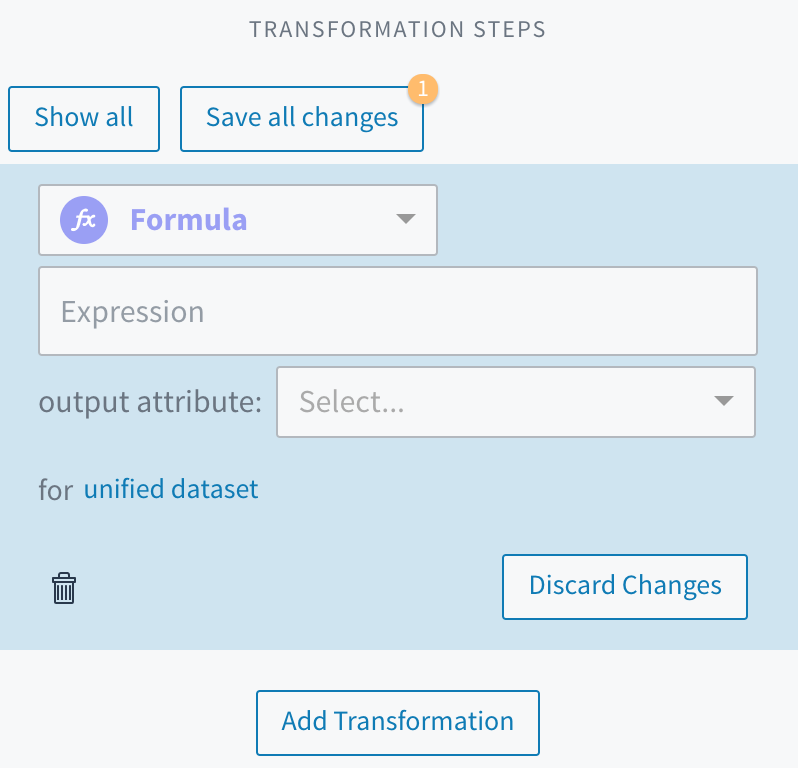# Formulas

Use can use the Formula option to quickly add a single expression in a well-formed `SELECT` statement.

Formulas are a tool for quickly writing scripts that operate on values within one column and access values in other columns. A formula offers a structured option for adding simple transformations, Formulas and scripts use the same building blocks, expressions(which may include functions), so you can use formulas to express many transformation scripts that begin with `SELECT *`.

# Formula or Script?

The following image illustrates how you can use either a formula or a script to complete the same transformation: converting the values in the "name" column to uppercase and populating the "name_cleaned" column with the resulting values.

When you use the Formula option to make this transformation:

• Tamr supplies the `SELECT *,` statement
• You supply the expression, which consists of a function `upper()` and the attribute you want the function to act on, `name`
• You select the output column, `name_cleaned`, from a dropdown list of the dataset's attributes

Alternatively, when you use the Script option you apply the required syntax as you type in each of these components. The result of using this formula or this script is identical.

# Tips for Formulas

Common use cases for Formula are concatenation, conditional logic, arithmetic operations, and replication of part of another column or an entire column.

The following tips discuss functions which you may find useful when creating formulas.

The default data type for all columns in the unified dataset is arrays of strings, even if they contain only one value. Therefore, the array functions that are available in Tamr can help you handle transformations correctly. The following is a list of useful array functions:

• `array()` converts a string into an array. This allows you to keep columns consistently typed. See array().
• `len()` is useful when writing `case` expressions and allows you to handle different array lengths. See len(). Note that this function differs from the `length()` function, which returns the length of a string.
• `get()` allows you to access a particular index of a multi-value field. For example, `get(<attributeName>, 1)` returns the second value from an array. See get().
• `to_string()` converts the input value to a string, including converting an array containing only one value to a string. For example, `to_string(<attributeName>)`. This is the preferred way to access strings from a single-value field. See to_string().
• `array_concat()` allows you concatenate array values into a string. Array concatenation adds values to an array in such a way that `array_concat(["A", "B"])` and `array_concat(["AB"])` both end up as `"AB"`. See array_concat().
• `array.concat()` allows you concatenate array values into a combined array. Array concatenation adds values to an array in such a way that `array.concat(['A', 'B'], ['CD'])` becomes `['A', 'B', 'CD']`. See array.concat().

The output attribute is the one to which output is written. Note that the transformation will overwrite any values already in that attribute. Attribute names are case sensitive in Tamr formulas and scripts, but statements, function names and keywords, such as `case`, `when`, and `empty` are not.Updated 10 months ago

### What's Next

 Working with Expressions Functions Writing Transformation Scripts

# Formulas

Use can use the Formula option to quickly add a single expression in a well-formed `SELECT` statement.

### Suggested Edits are limited on API Reference Pages

You can only suggest edits to Markdown body content, but not to the API spec.